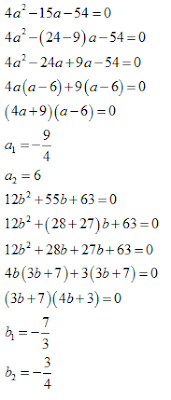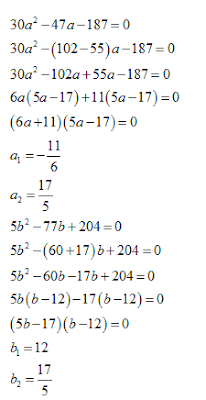New Students Offer - Use Code HELLO

# Quadractic Equations For IBPS PO: Part 5

1. In the following question two equations (A) and (B) given. You have to solve both:
I. 4a² -15a - 54 =0
II. 12b² +55b +63 = 0

A. If a > b
B. If  a < b
C. If  a = b
D. If  a < b
E. If  a > b

2. In the following question two equations (A) and (B) given. You have to solve both:
I.
7a² - 37a +10 = 0
II.  b² +10b +21 = 0

A. If  a < b
B. If  a = b
C. If  a < b
D. If  a > b
E. If  a > b

3. In the following question two equations (A) and (B) given. You have to solve both:
I.
3a² + 17 √3a + 72 = 0
II.  b² + 1b - 30 =0
A. If  a= b
B. If  a < b
C. If  a > b
D. If  a > b
E. If  a < b

4. In the following question two equations (A) and (B) given. You have to solve both:
I.
30a²  - 47a - 187 = 0
II.  5b²  - 77b + 204= 0
A. If a <
B. If  a > b
C. If  a > b
D. If  a < b
E. If  a = b

5. In the following question two equations (A) and (B) given. You have to solve both:
I.
a² -841 = 0
II.  b³ - 6859 = 0
A. If a > b
B. If  a > b
C. If  a < b
D. If  a = b
E. If  a < b

#### 1. Ans. C.

Solution:#### 2. Ans. D.

Solution:#### 3. Ans. A.

Solution:#### 4. Ans. D.

Solution:#### 5.  Ans. D.

Solution: